Search Algorithms Sequential Search Linear Search Binary Search

• Slides: 18Search Algorithms Sequential Search (Linear Search) Binary Search Data Structures Using C++ 1Sequential Search O (n) l. A sequential search of a list/array begins at the beginning of the list/array and continues until the item is found or the entire list/array has been searched Data Structures Using C++ 2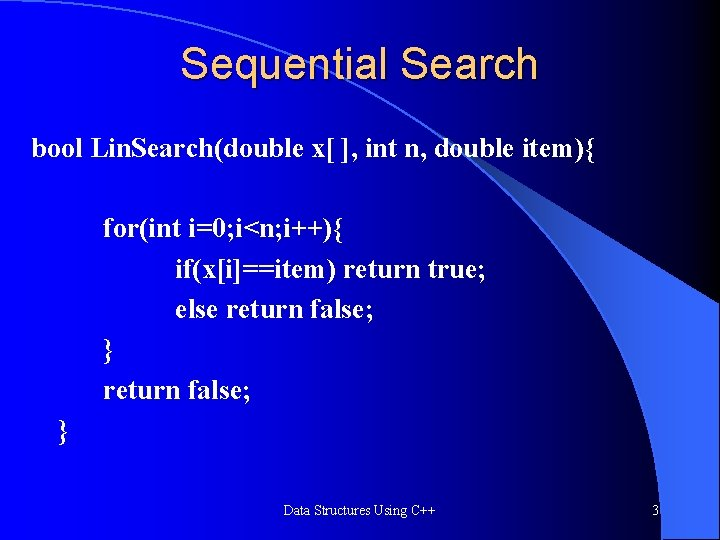Sequential Search bool Lin. Search(double x[ ], int n, double item){ for(int i=0; i<n; i++){ if(x[i]==item) return true; else return false; } Data Structures Using C++ 3Search Algorithms Suppose that there are n elements in the array. The following expression gives the average number of comparisons: It is known that Therefore, the following expression gives the average number of comparisons made by the sequential search in the successful case: Data Structures Using C++ 4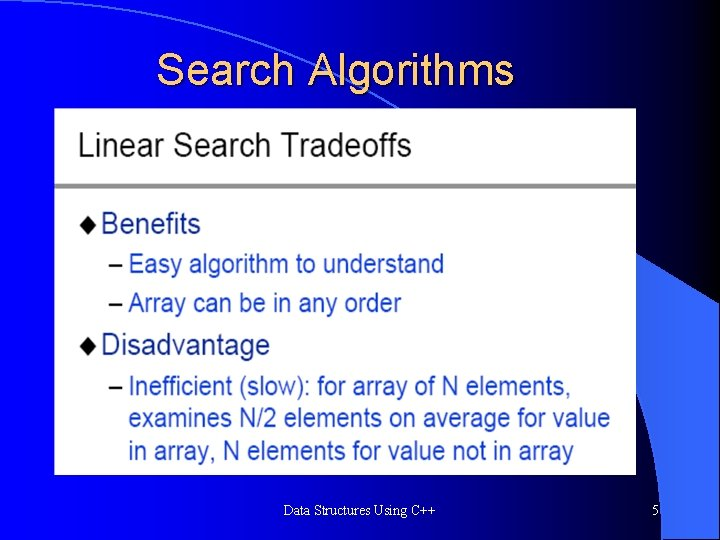Search Algorithms Data Structures Using C++ 5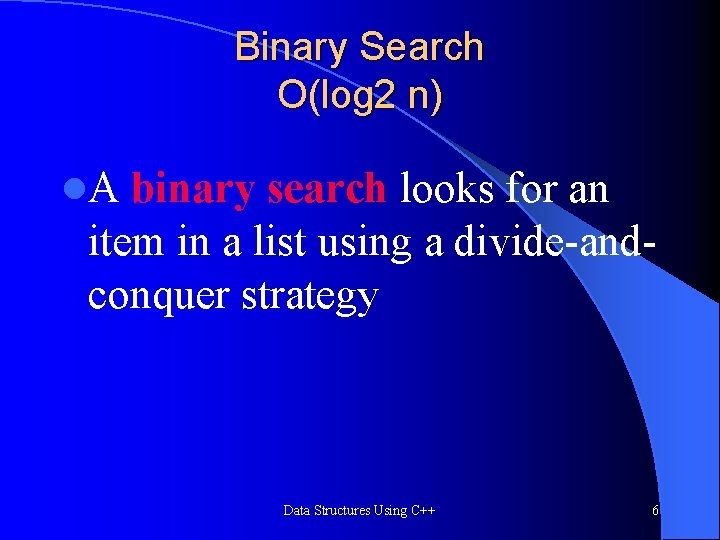Binary Search O(log 2 n) l. A binary search looks for an item in a list using a divide-andconquer strategy Data Structures Using C++ 6Binary Search – Binary search algorithm assumes that the items in the array being searched are sorted – The algorithm begins at the middle of the array in a binary search – If the item for which we are searching is less than the item in the middle, we know that the item won’t be in the second half of the array – Once again we examine the “middle” element – The process continues with each comparison cutting in half the portion of the array where the item might be Data Structures Using C++ 7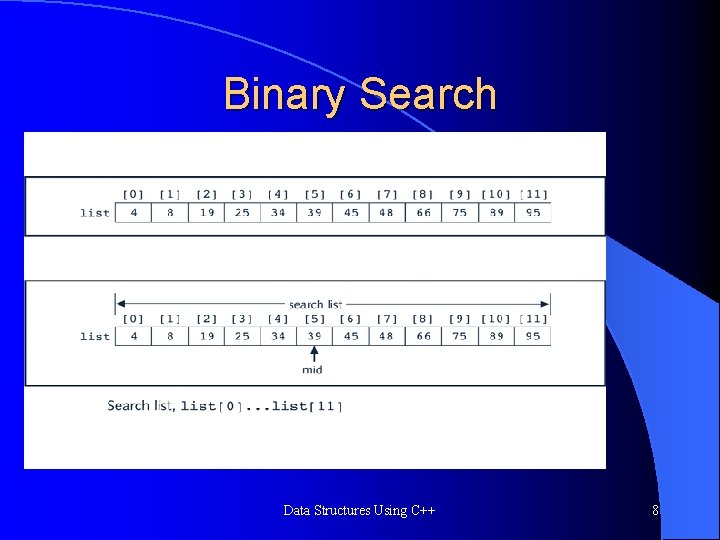Binary Search Data Structures Using C++ 8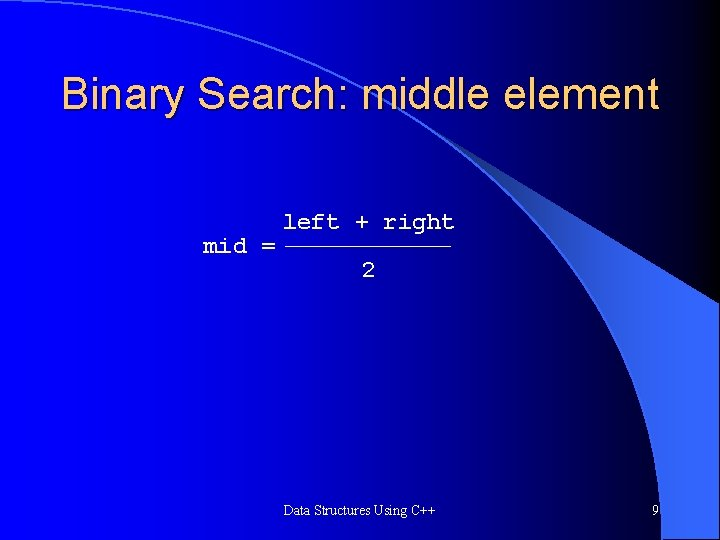Binary Search: middle element mid = left + right 2 Data Structures Using C++ 9Binary Search bool Bin. Search(double list[ ], int n, double item, int&index){ int left=0; int right=n-1; int mid; while(left<=right){ mid=(left+right)/2; if(item> list [mid]){ left=mid+1; } else if(item< list [mid]){right=mid-1; } else{ item= list [mid]; index=mid; return true; } }// while return false; Data Structures Using C++ 10Binary Search: Example Data Structures Using C++ 11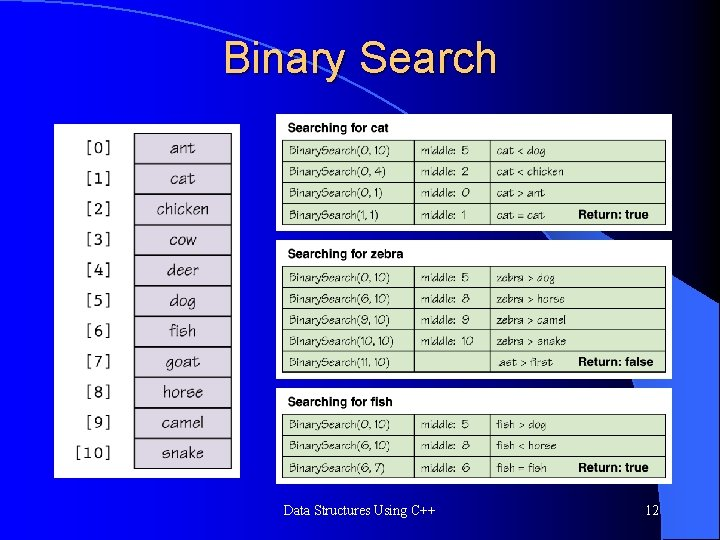Binary Search Data Structures Using C++ 12Binary Search Data Structures Using C++ 13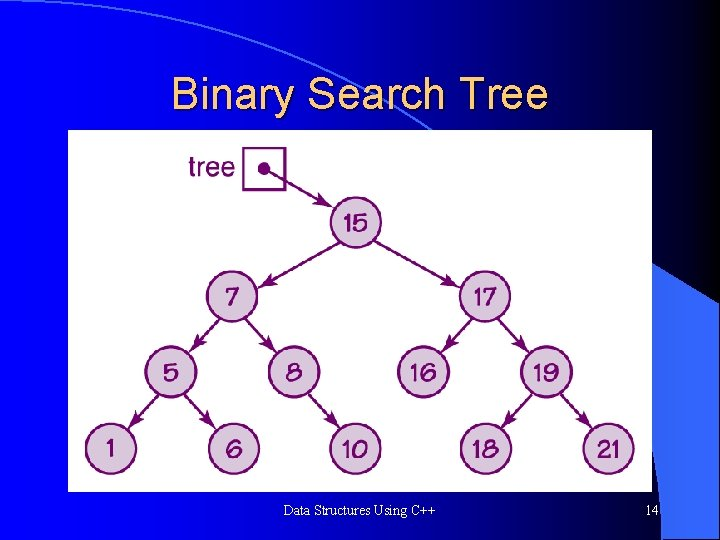Binary Search Tree Data Structures Using C++ 14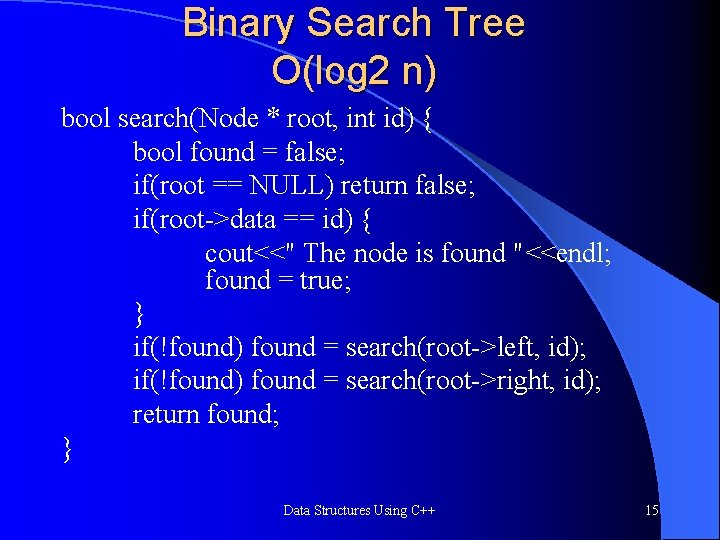Binary Search Tree O(log 2 n) bool search(Node * root, int id) { bool found = false; if(root == NULL) return false; if(root->data == id) { cout<<" The node is found "<<endl; found = true; } if(!found) found = search(root->left, id); if(!found) found = search(root->right, id); return found; } Data Structures Using C++ 15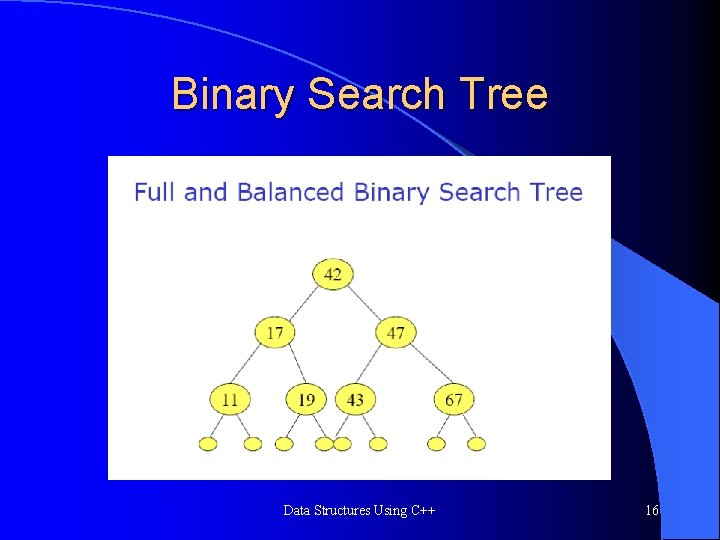Binary Search Tree Data Structures Using C++ 16Binary Search Tree Data Structures Using C++ 17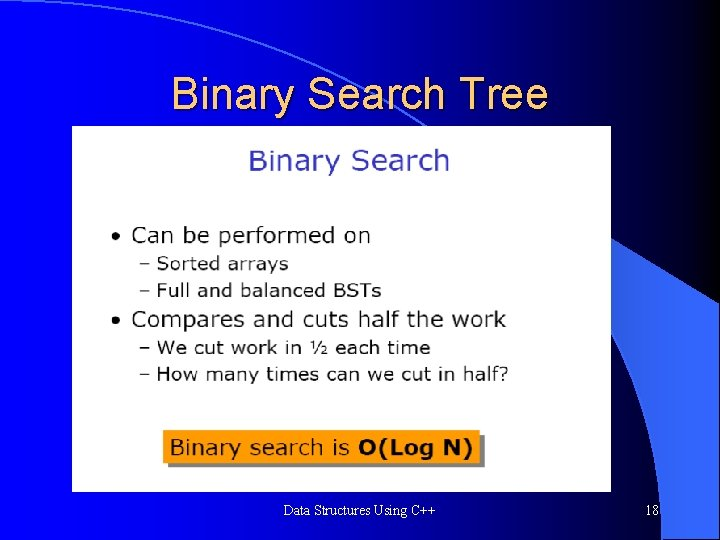Binary Search Tree Data Structures Using C++ 18